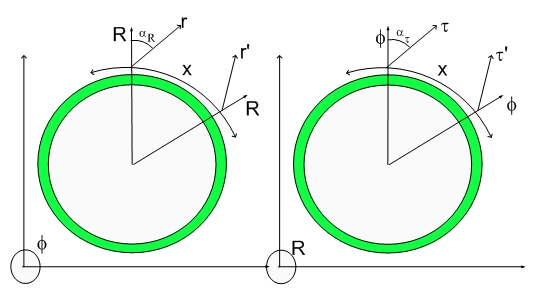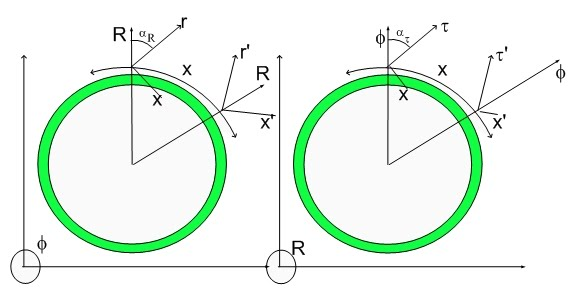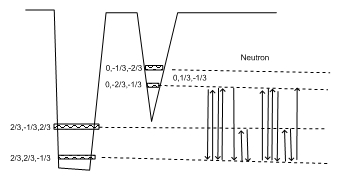# ReadMe!!!!

Viewing posts from June, 2008

## Sir Isaac Newton - The AlchemistSir Isaac Newton - The Alchemist

Sir Isaac Newton introduced the concept of Gravitation as a pervasive force controlling the motion of all Celestial and Earth bound objects. Later in life, Newton redirected his attention to Alchemy.

At his time, it was easy to think that the Ancients- who had such a rich culture - had many of their teachings lost in the night of times (Dark Ages) ... and they were.... until the Renaissance... I am sure many of their achievements are still not fully recovered.

Newton realized that there is such a thing as Action-At-Distance (in the proper relativistic obeying physical sense). It turned out that there is only action at distance, nothing else. Even when two surfaces are in contact, there is a distance between the layers of atoms that comprise those surfaces.

Newton correctly understood that there should be a framework of waves guiding everything that happens in this Universe. He looked into the hairlike growth of crystals in solution and envisioned a field guiding the positioning of each atom or piece of matter...:)

He was very close to understanding the whole Universe. The only missing element was the fourth spatial dimension and the understanding of how fine was the framework.

In my theory, I proposed that this framework is a mesh of dilatons with a four-dimensional wavelength equal to the Compton wavelength of a Fundamental Dilator (a Hydrogen atom)...:)

In the derivation of my Grand Unification equations I used the dilator and the dilaton field created by it as a single entity. This is because a dilator surfs its own dilaton field as it creates it or the local dilaton field if it is interaction with other dilators. Of course, the dilaton field extends aways even in our 3D spatial hypersphere as it is created and has its own existence.

The 4DSpacetime projection of the 5D Spacetime dilaton field is the de Broglie wave associated with the particle.

This means that the de Broglie waves are not just an artifact nor are they matter waves in the current sense. Dilators (matter) still are localized within the de Broglie wavefront. The difference is that given a mechanism for de Broglie wave interference, the matter (dilators) will follow trajectories that reflect the interference pattern. Given enough dilators, the complete resulting interference deposition pattern will occur.

The dilator free dilaton field (matter free metric) will contain all the oscillating metric deformations in accordance to my Grand Unification Equation - the equation for the dilaton field resulting from a group of dilators.

As you know, one can only guess what Newton was trying to see when he recreated crystal growth in solution. Those experiments yield interesting and well defined structures which are the result of atomic interaction...

I imagined the curious Newton trying to see them as the result an Action-At-Distance force. In the Hypergeometrical Universe language, he would be trying to see the effect of a dilaton field.

Since the dilaton field is simple to understand in a four dimensional spatial framework but very difficult to understand just by looking at their hyperbolic projections, Newton faced a very difficult challenge.

Newton's alchemical crystal growing experiments reflected that dilaton field in an much more complex manner. Those experiments did not provide the proper insight but they were a fair attempt at discovering dilatons.

Newton arrive too early but he had the enough insight to keep looking for the dilaton framework. I benefited from Einstein's mistakes like somehow thinking that only Gravitation deformed space...:) Why would that be the case????

Sheep-like scientists have been repeating this Mantra for a hundred years without ever asking that simple question.

More than three hundred years ago Sir Isaac Newton asked it and pursued its answer in his Alchemical Experiments. That is what I would call the Wisdom of the Ancients.

Cheers,

MP

## The Echoes of the Big BangThe Big Bang and its Echoes...:)

The obvious question to ask is if matter didn't, doesn't and will never travel faster than the speed of light, what generated the echoes..>:)

## The Mass of a NeutrinoThe Mass of a Neutrino

Einstein's paradigm of a 4D Spacetime was later patched up with compact curled spaces - one for each new unnecessary quantum number (Strangeness, Bottom-Up etc) scientists introduced into their Standard Model...:)

The resulting patched Spacetime is still basically a 4D Spacetime in nature, that is, if there is a 3D point where the Universe started (Big Bang), then that same universe can return (Big Crunch) under the force of Gravitation...:)

That Big Crunch depends upon the sum of all masses and that includes those ubiquitous neutrinos...:)

Much speculation, calculations etc has been done on that subject.

Let me tell you my view of it...:)

As I mentioned in the Galileo Biggest Blunder, inertial mass and Gravitational Mass have been inadvertently made equal in the Galileo' Equivalence Principle.

In my theory that is not the case. Inertial and Gravitational Masses are only the same for standard matter...:) stuff composed of protons, electrons and neutrons.

Inertial mass is associated with the resistance to deformation of Fabric of Space on the footprints of matter (dilators).

Gravitational mass is modeled in my theory as the 4D displacement volume associated with dilator's cycles. This means that one can have a footprint on the 3D Fabric of Space and have ZERO GRAVITATIONAL MASS. This will always be the case if the frequency of the dilaton fields are not the same as the fundamental dilator frequency.

Every time someone says something about being in phase or having a frequency one has to mention what kind of clock is being used. Of course, proper time is not a good clock...;) The Tau vector (direction of Proper Time) depends upon local deformation of the Fabric of Space. This makes it clear that the only clock one can use is the Cosmological Time which is visible in both cross-sections of the Lightspeed Expanding Hyperspherical Universe shown above.

To understand what one means by frequency just analyse the Neutron Coherence Diagram below:One can easily recognize the Electron and Proton sub-coherences. The remaining steps correspond to rotations within the 3D space. Notice that the two states involved (2/3,-1/3,2/3) and (2/3,2/3,-1/3) differ just by a 90 rotation around the x axis. Four rotations will bring the deformational state back into its original state.

## Pion Decay DetailsPion Minus Decay Details

I should repeat the same detailed description I created for the Delta decay.

A complex coherence sequence with multiple fundamental dilator chords is a polymer, in the sense that its 3D representation seemingly contains more than one particle. In reality, one should be able to see three particles (does that rings a Bell someplace) in the case of a Pion Minus, two in the case of a Neutron...

The decaying reaction for the trimeric Pion Minus can be redrawn as:

## Pion Minus DecayRSS / Atom